## Introduction

Written Arithmetic supports the addition of two or three numbers. These numbers can be integers or decimal numbers.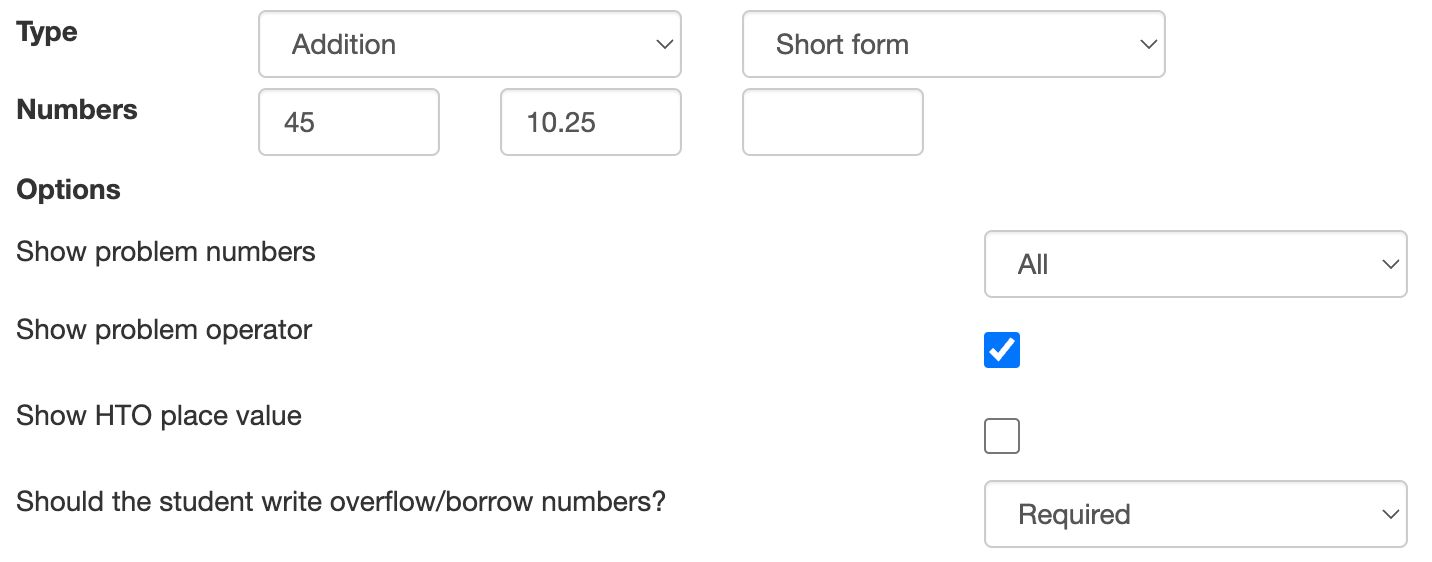Figure: Configuring an addition problem.

Note

Written Arithmetic writes decimal numbers with a decimal dot or comma, depending on the student profile. However, the author should always use the decimal dot.

## Short and extended forms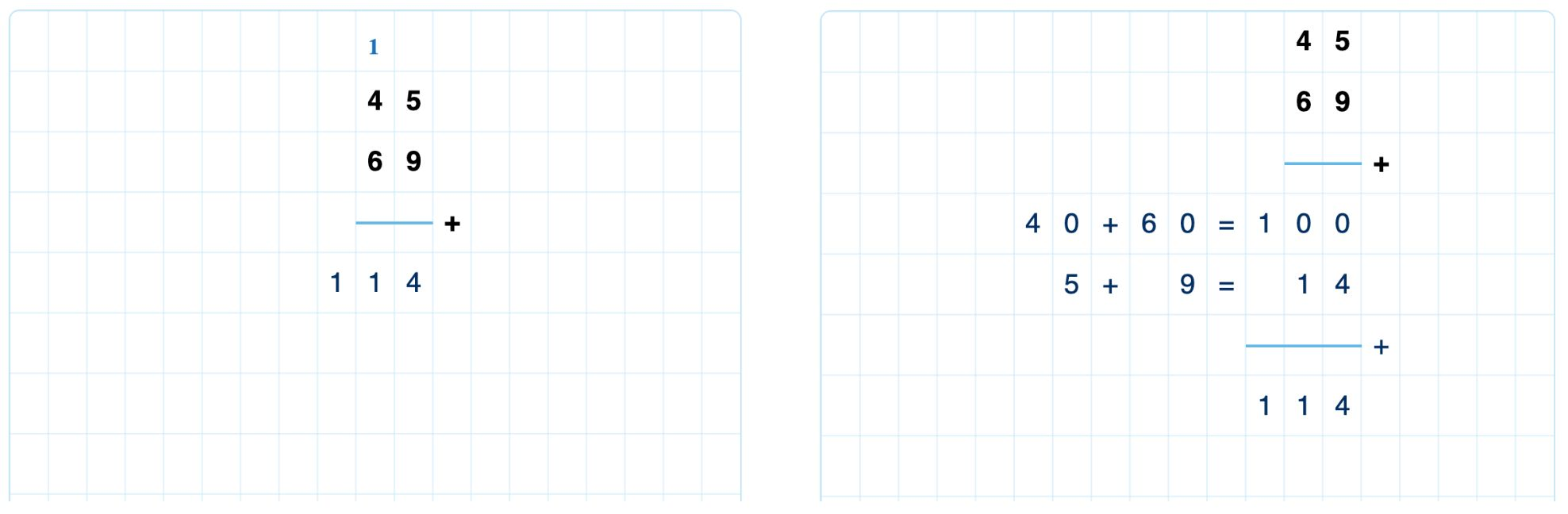Figure: Short and extended forms for addition.

You can specify different calculation forms. The short form is the most compact form, using a carry to move overflows to the next place value. The extended form is a more intuitive approach where the ones, tens, hundreds, etc., are added separately. You can also let students decide what form to use.

Note

The extended form does not support the addition of decimal numbers.

If you choose the short form, you can decide if students must write the carries or if they can omit them. Likewise, in the extended form, you select if students must write the complete calculation for each place value or if it is ok to write their sums.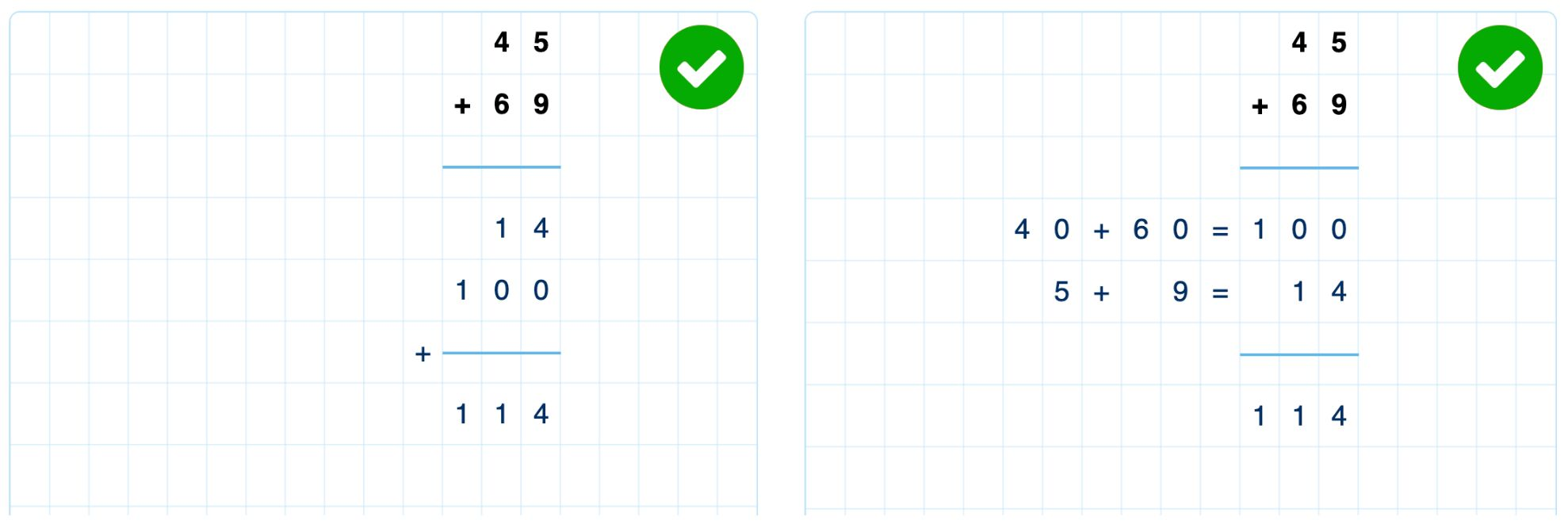Figure: Extended forms with and without the calculation for each place value. Note the different order of the partial calculations.

In the extended form, students can choose the order of the place value calculations. For example, they can add the ones first, followed by the hundreds and the tens. You can also force students to write the place value calculations in a fixed order (ones first, then the tens, etc.) or a consistent order (low to high or high to low).

## Choose what is visible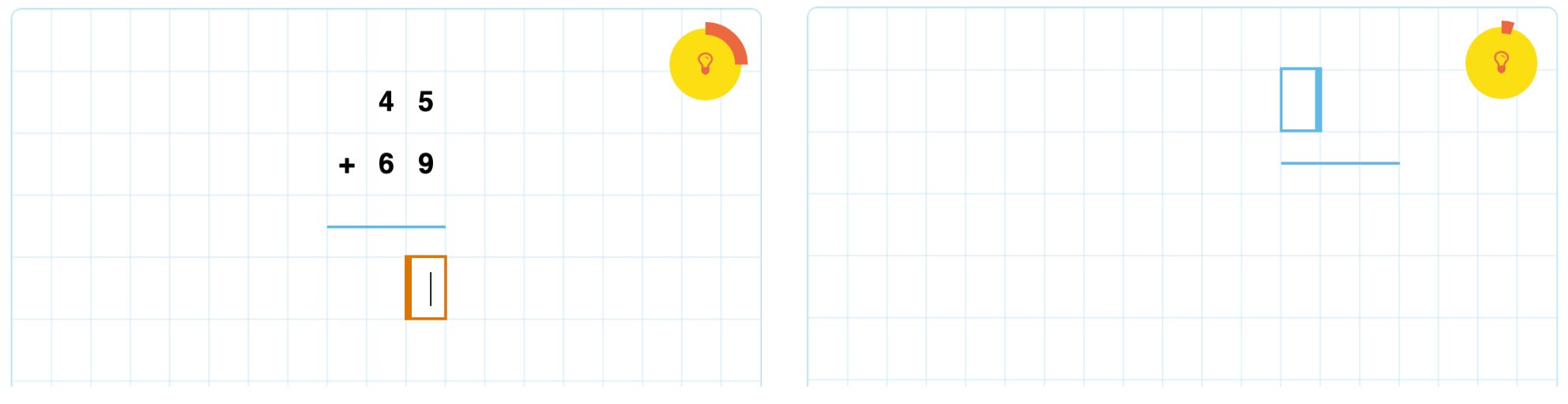Figure: Problem visible or hidden at question start. The line is always visible.

When the question starts, you configure what parts of the problem are already visible. For instance, you choose to show only one of the numbers and hide the addition operator. If the problem values are absent, students can write them in any order. The line is always present.

You can choose what is visible in the place value calculations in the extended form. There are four options:

1. Nothing is visible
2. Only the operators ('+' and '=') are visible
3. The operators are hidden but show up automatically when the cursor is near
4. The full calculations are visible.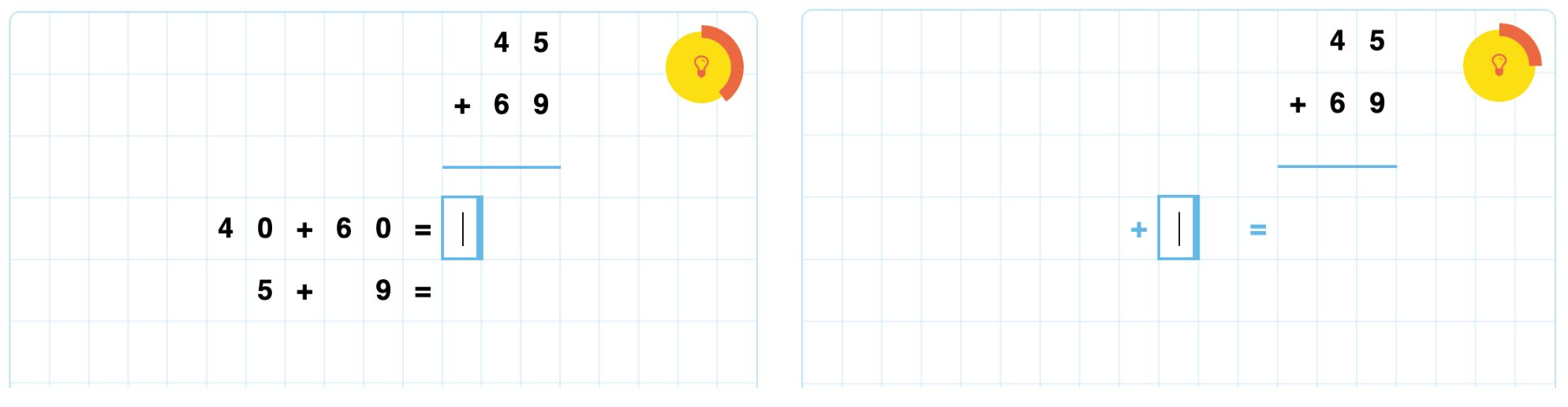Figure: Show full place value calculations or automatically show the operators.

## Place value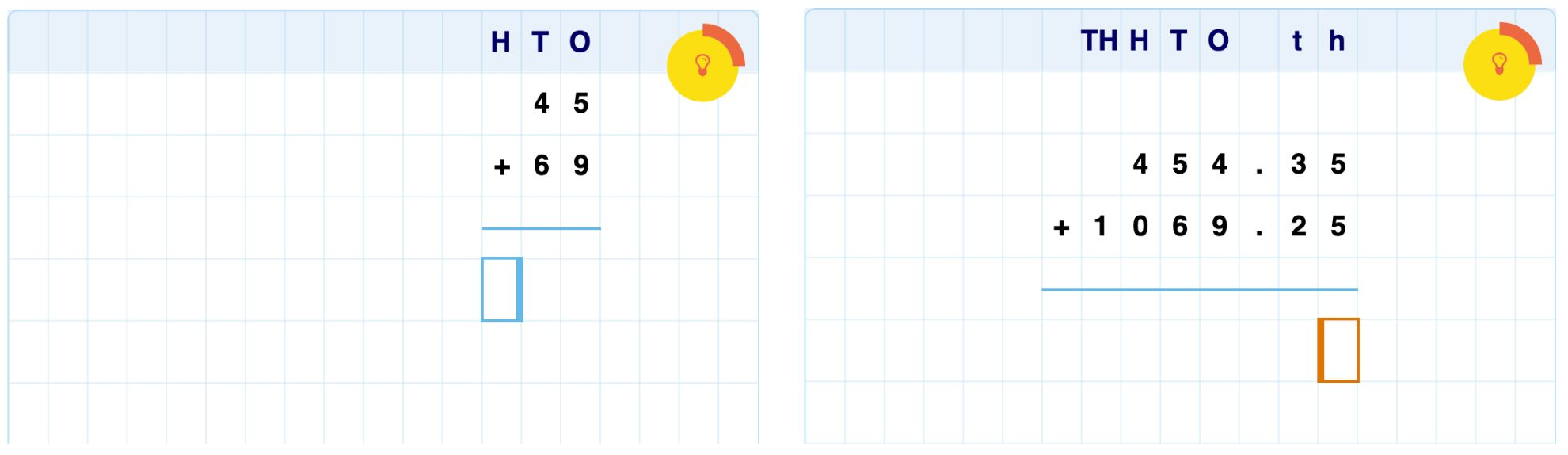Figure: The place value ribbon adjusts to the values in the problem.

You can include a ribbon with place value indicators. In English, this banner shows the symbols H, T, and O, representing Hundreds, Tens, and Ones. More symbols are automatically added if required.